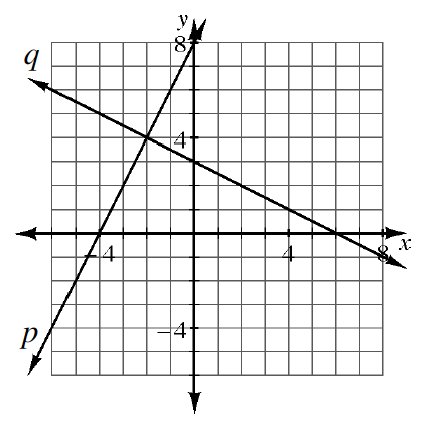### Home > INT1 > Chapter 6 > Lesson 6.4.2 > Problem6-136

6-136.The graphs of two equations are shown at right.

1. Write an equation for each line.

Use$y = mx + b$. Notice that line $q$ is negative and the slope is not steep.

$\text{Line }p: \ y=2x+8$

$\text{Line }q:\ y=-\frac{1}{2}x+3$

2. Does $x=-2$ and $y = 4$ solve both of the equations you wrote in part (a) above? How can you tell without actually checking the solution in the equations?

Notice that the product of the slopes is $-1$. What does that mean about the lines?

3. What is the relationship between the two lines in part (a)? How do you know?

Where is the point $(-2,4)$ on the graph? How does it relate to the graph?

4. Solve the system of equations you wrote in part (a) algebraically. Verify that your solution matches the one shown in the graph.

To solve the system, begin by using the Equal Values Method.

Eliminate the fraction by multiplying each term by $2$, then solve for $x$. Substitute $x$ into the equation for line $p$ and solve for $y$.
Check both values in the equation for line $q$.